# Lesson 3 – Further elements of the kinetics of rigid bodies

1.16. Trusses
1.16.1. Planar trusses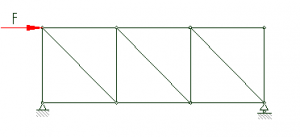• Consists of straight rods
• The rods are connected by joints
• Loaded or supported on the joints only (only at the ends of the rods, never in between!)
• External statical determination: n_b+n_k=s
• Internal statical determination: r=2*c-3  Where r: the number of rods and c: the number of joints
• The weight of the structure is neglected

Because load is applied only at the nodes, only rod-direction forces act in the bars. The unloaded rods are called blind rods.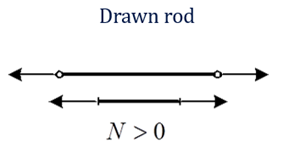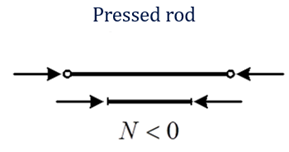1.17 FRAMES
Three-hinge frames: structures that are internally not stabile, they would collapse without supports.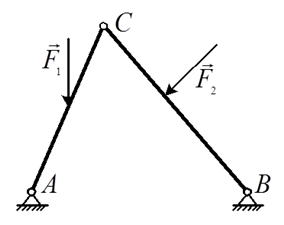Example:
Given: Dimensions and load of the structure

Forces in y direction can be determined by examining the whole structure. But not in x direction!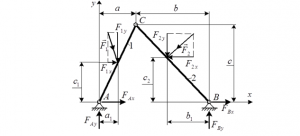Forces in y direction are calculated using moment equations like so: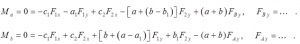1.18 FRICTION

Friction is a resistive force acting between two contacting surfaces opposite of the direction of forces parallel to the contact surfaces. Friction always acts in a way to prevent movement.

1.18.1 THE COMPONENTS OF FRICTION

If the atoms of the two contacting surfaces can get close enough to each other, a weak attractive force acts between them, thus producing a resistance when the surfaces try to move along each other. This component of friction is emphasized in the case of relatively smooth surfaces.

• Deformation
In case of higher surface roughness, when two surfaces move along each other, small bits of pieces break down or are deformed on them. This deformation energy is lost in a form of resistance against movement.

A good example to demonstrate low and high roughness friction: pole vs. rope climbing.

These two components are always present in different ratios, depending on the roughness of both contacting surfaces

1.18.2 IS THE FRICTION GOOD OR BAD?

In some cases, friction is harmful, or is meant to be reduced:
• Wear, abrasion
• Losses, resistances

In engineering, when designing structures with moving parts, friction is like an arch enemy, as it greatly determines the efficiency of the designed machinery.

Many times, friction is useful or even necessary:
• Walking
• Breaking, starting
• Everyday life…
Just imagine what would happen without friction. Every unfixed object and creature would end up in lowest points of Earth’s surface unable to move whatsoever.

1.18.3 FRICTION FORCE:
When a force parallel to the contacting surfaces (pulling force from now on) is applied to a body, the friction force acts in the contact point.
If the pulling force increases, the friction force increases as well – up to a maximum value. This is the static friction force.
Then when the body is moving, the friction force resisting the movement is lower than the static friction force. This is called the dynamic friction force.
As this paragraph is about statics, we only discuss the case of static friction and especially the boundary condition of movement.

Friction force depends on:
• Roughness of both surfaces
• The magnitude of the forces compressing the surfaces together
It does NOT depend on:
• The size of the surfaces

1.18.4 THE FRICTION COEFFICIENT

Definition: μ: The ratio between the normal and the maximum friction force is called the friction coefficient. It depends on the physical parameters of the contacting surfaces. It is a dimensionless value, as it is the ratio of two forces.

Thus,

Fs =μ*FN

But only in the boundary state of sliding!

Where:

Fs: friction force

FN: normal force compressing the surfaces together

μ: friction coefficient

1.18.7 REDUCTION OF FRICTION FORCE

In engineering, friction needs to be reduced very frequently. There are different methods to do so.

Appropriate roughness: Choosing both the surfaces roughness in a way that minimizes friction.

Bushing, coating: Placing a third material between the two contacting surfaces that can slide more easily or is cheap to be replaced when worn.

Lubricant: Similar to bushing and coating, but this time the third material is not quite solid. Oils and greases are widely used as lubricants, their purpose is to keep the contacting surfaces away from each other, preventing contact.

Rolling (wheels, bearings): Eliminating sliding entirely, wheels are used to roll on one of the surfaces, carrying the other object, or enable rotation of a shaft within a bore.

Magnetic levitation: Keeping the surfaces apart using magnets results in changing the friction of the surfaces to only air resistance, which is a lot lower in most cases.